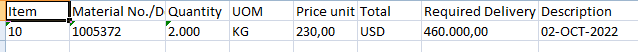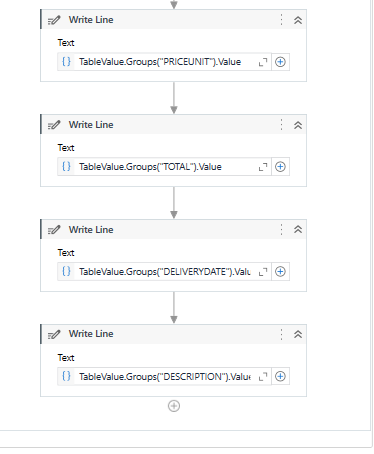# Extract Value using Reg Exr

Hello

I have extract value PO number and date using Reg ex.
How to extract?

example:

PO number/date AEROPORTO - - MG 37031-090
4500617273 / 15.07.2022 61.190.096/0016-79
Contact person/Telephone 345678990
Douglas Cavalcante/2345-3421 Your vendor number with us:
Our fax number

out put:

1. 4500617273
2. 15.07.2022

Thanks
minal patil

1 Like

Hi,

``````m = System.Text.RegularExpressions.Regex.Match(yourString,"(?<PO>\d+)\s+/\s+(?<DATE>\d{2}\.\d{2}\.\d{4})\s+")
``````

Then

``````m.Groups("PO").Value
m.Groups("DATE").Value
``````

Sequence.xaml (5.4 KB)

Regards,

@minal.patil

Let us assume the string is in a variable str

To get po number use

`PO = system.text.regularexpressions.regex.match(str,"(?<=PO number.*\n)\d+").value`

Then ti get date us the po number as an anchor and get it like below

`dateval = system.text.regularexpressions.regex.match(str,"(?<=" + PO + " \/ )\d{2}\.\d{2}\.\d{4}").value`

In this PO is the variable extracted before

Cheers

1 Like

Thanks it’s working

AND ONE MORE
INPUT:
3456-23459 Manufacturer: - –

D No 8-8-123, ROAD No, 36-1-34
Vendor: DR.MINAL GULABRAO LIMITED PATIL XYZ - IN

OUT PUT:

1. D No 8-8-123, ROAD No, 36-1-34
2. DR.MINAL GULABRAO LIMITED PATIL XYZ - IN

Hi,

Can you try the following?

``````System.Text.RegularExpressions.Regex.Match(yourString,"(?<=Vendor address:\s+).*").Value
``````

Vendor

``````System.Text.RegularExpressions.Regex.Match(yourString,"(?<=Vendor:\s+).*").Value
``````

Regards,

1 Like

thanks it is solve

1 Like

Input:

Item Material Description Qty Unit Price per unit By Net
value
Ncm Code

## 00001 H.100138.60034 CLORIDRATO SERTRALINA (C1) 880 Kilogram 250,00 1 220.000,00 29214990 Deliv.date Day x.y.z Manufacturer: xyz Manufacturer Adress: ---------------------------------------------------

00002 H.100138.60034 CLORIDRATO SERTRALINA (C1) 970 Kilogram 250,00 1 242.500,00
29214990

output:

1. 00001 and 00002(all item no. in table)
2. H.100138.60034 and H.100138.60034 (all material in table)
3. CLORIDRATO SERTRALINA (C1) and CLORIDRATO SERTRALINA (C1) (all Description in table)
4. 880 and 970 (all qty in table)
5. Kilogram and Kilogram (all unit in table)
6. 250,00 and 250,00 (all Price per unit in table)
7. 1 and 1 (all by in table)
8. 220.000,00 and 242.500,00 (all net in table)
9. 29214990 and 29214990 (all ncm code in table)

Give it a try.

``````mc = System.Text.RegularExpressions.Regex.Matches(yourString,"(?<ITEMNO>\d+)\s+(?<MATERIAL>\S+)\s+(?<DESCRIPTION>.+?)\s+(?<QTY>[.,\d]+)\s+(?<UNIT>[A-Za-z]+)\s+(?<PRICEPERUNIT>[.,\d]+)\s+(?<BY>\d+)\s+(?<NET>[.,\d]+)\s+(?<NCMCODE>\d+)")
``````

Sequence.xaml (8.8 KB)

Regards,

Thanks For help.
it is solve

1 Like

some problem is occur. when i am scrap vendor address using this reg exp the output value is blank/null

Hi,

Probably there is something different with the above sample. You need to modify pattern for it.

Regards,

OK

After scraping:-

Item Material No./ Quantity UOM Price Unit Total Required
Description Delivery Date

10 1005372 2.000 KG 230,00 USD/1 KG 460.000,00 02-OCT-2022
Clopidogrel Bisulphate Form 1

Reg. Exp:-

(?\d+)\s+(?\S+)\s+(?[.,\d]+?)\s+(?[A-Za-z]+)\s+(?[.,\d][A-Za-z]+)\s+(?[.,\d]+)\s+(?[.,-\d][A-Za-z]+)\s+(?.+?)\s

This is correct or not

Incorrect, as the following.

You can check if your pattern is valid at online regex test site such as regex101, regexstorm etc

The pattern will be like the following, for example.

``````(\d+)\s+(\S+)\s+([.,\d]+?)\s+([A-Za-z]+)\s+([.,\d]+)\s+([A-Za-z]+).(\d)\s([A-Za-z]+)\s+([.,\d]+)\s+(\d+-[A-Za-z]{3}-\d+)\s+.+
``````

However I recommend to use Named group in order to handling matched result easier.

Regards,

Using above Reg exp out put is :But

Expected Output is:

in for loop we use :Hi,

Please tune position of parenthesis. For now, give it a try the following.

``````(\d+)\s+(\S+)\s+([.,\d]+?)\s+([A-Za-z]+)\s+([.,\d]+\s+[A-Za-z]+.\d\s[A-Za-z]+)\s+([.,\d]+)\s+(\d+-[A-Za-z]{3}-\d+)\s+.+
``````

Regards,

Thanks it solve

when i use this reg exp tester it gets proper out put but when i run that time it will get blank output.

Input:
Vendor Contact Information
Dr. Minal’s Laboratories SA Contact: xxx yyy
Maharashtra 12 . Email: xxx.yyy@zzzz.com
4051 Pune
India

Contact: xxx yyy

OutPut:

1. Dr. Minal’s Laboratories SA
2. Maharashtra 12
4051 Pune
India

It’s necessary 2 regex. Give it a try the following.

Maharashtra 12

``````.*(?=Email:)
``````

4051 Pune
India

``````(?<=Email:.*\r?\n)[\s\S]+?(?=Contact:)
``````

Regards,

Thanks

can you give me link of study material of Reg exp.?

HI,

How about the following as first step?

Regards,